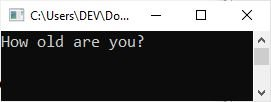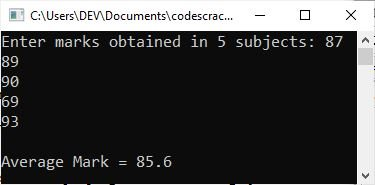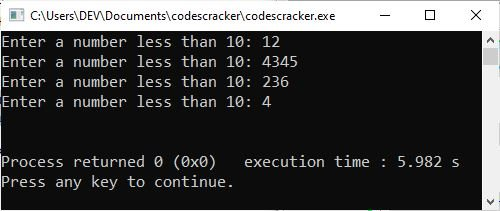# C++ Variables

Variables, one of the most important concepts in the C++ programming language, will be introduced and investigated in this article. So, without further ado, here is a list of topics that are all related to the "variables" discussed in this article.

## Introduction to C++ variables

Variables are storage locations with names that can be changed while the program is running. For example, to store a student's name and grades during a program run, we need two storage locations with different names so that they can be easily distinguished.

Variables, called "symbolic variables," serve the purpose. The variables are called symbolic variables because these are named locations. For instance, the following statement declares a variable i of the data type int:

`int i;`

A separate (next) article describes data types. To summarize, when declaring a variable in a program, you must specify its data type to tell the compiler what type of data the variable can store. For example, since the above variable "i" was defined using "int,", then that variable can only store integer values.

### Values associated with a symbolic variable in C++

There are the following two values associated with a symbolic variable:

• Its data value is stored at some location in memory. This is sometimes referred to as a variable's rvalue (pronounced "are-value").
• Its location value, that is, the address in memory at which its data value is stored, This is sometimes referred to as a variable's lvalue (pronounced "el-value").

## Declaration of a Variable in C++

Here is the general form to declare a variable in C++:

`type variableName;`

Here, type is any valid C++ data type, and variableName is the variable's name. An identifier is a variable name. As a result, when declaring the name of a variable, all of the rules of identifier naming apply. The following declaration declares an int variable age:

`int age;`

Consider the following program as an example.

```#include<iostream>
using namespace std;
int main()
{
int age;
cout<<"How old are you? ";
cin>>age;
cout<<"\nYou're "<<age<<" years old.";
cout<<endl;
return 0;
}```

The following snapshot shows the initial output produced by the above C++ program:Now supply your age, say 19, and hit the "ENTER" key to produce the following output:To declare a signed or unsigned variable, place these modifiers before the data type. For example:

```signed int value;
unsigned short count;
signed long gross;
double pival;
long double res;```

### Declare multiple variables at the same time in a single statement in C++

To declare more than one variable of the same data type in a single statement, follow the following general form:

`type variable1, variable2, variable3, ..., variableN;`

For example:

`double salary, wage;`

## Initialization of a variable in C++

Declaring a variable without a value can be called an "uninitialized variable," and the variable's value is said to be "undefined." A first value (initial value) may be specified in the definition of a variable. A variable with a declared first value is said to be an initialized variable.

The following is the general syntax for assigning values to variables in C++.

`type variableName = value;`

Here is a code fragment showing the variable initialization in C++.

`int val = 100;`

Let's take an example program demonstrating C++ variable initialization.

```#include<iostream>
using namespace std;
int main()
{
int a = 10, b = 20;
cout<<"The value of 'a' = "<<a;
cout<<endl;
cout<<"The value of 'b' = "<<b;
cout<<endl;
return 0;
}```

The output produced by the above C++ program should exactly be:

```The value of 'a' = 10
The value of 'b' = 20```

However, instead of initializing a variable's value when it is declared, you can do so later in the program. For example:

```int val;
val = 20;```

Let me create another example for your understanding. So the following program is created after modifying the previous program.

```#include<iostream>
using namespace std;
int main()
{
int a, b, sum;
a = 10, b = 20;
cout<<"The value of 'a' = "<<a;
cout<<"\nThe value of 'b' = "<<b;
sum = a+b;
cout<<"\n\nThe sum of 'a' and 'b' is "<<sum;
cout<<endl;
return 0;
}```

The following is the output:

```The value of 'a' = 10
The value of 'b' = 20

The sum of 'a' and 'b' is 30```

### Dynamic initialization of a variable in C++

The ability to initialize variables at runtime is another feature of C++. This is known as "dynamic initialization." Variables can be initialized at run time by using expressions in their declaration. For example, consider the following variable declaration:

```.
.
.
float avg;
avg = sum/tot;
.
.
.```

You can also combine the above two statements into one, like this:

`float avg = sum/tot;`

It will initialize "avg" using the information available at run time, i.e., using the values of "sum" and "tot" at run time. Here is an example program demonstrating dynamic initialization in C++.

```#include<iostream>
using namespace std;
int main()
{
float mark, sum = 0, tot = 5, avg;
cout<<"Enter marks obtained in 5 subjects: ";
for(int i=0; i<5; i++)
{
cin>>mark;
sum = sum+mark;
}
avg = sum/tot;
cout<<"\nAverage Mark = "<<avg;
cout<<endl;
return 0;
}```

The following snapshot shows the sample run of the above C++ program:In the preceding example, the variable "avg" was dynamically assigned at program runtime, whereas the variable "sum" was dynamically changed during program runtime.

Important: Variables that haven't been initialized aren't empty. If you do not initialize your variables, they will contain junk (or garbage) values left over from the program that last used the memory location they now occupy until the program places a value there.

## C++ Variable Scope

Variable scope represents the scope of a variable, that is, where it can be used in a C++ program. Basically, there can be two scopes for a variable in a C++ program, which are:

### C++ Local Variables

Local variables are those variables that are declared inside a function or block. Here is an example of local variables in C++, demonstrating the variable scope in C++:

```#include<iostream>
using namespace std;
int main()
{
while(true)
{
int num;
cout<<"Enter a number less than 10: ";
cin>>num;
if(num < 10)
break;
}
cout<<endl;
return 0;
}```

The following snapshot shows the sample run of the above C++ program with some user inputs:The above program continues receiving inputs until the user provides a number less than 10. But this is not the point; the point is, the variable "num" declared inside the "while" block is known only to that block. Therefore, if we try to access this variable outside the "while" block, then it will be unknown or undefined. So the variable "num" can be called a local variable.

### C++ Global Variables

Global variables are variables that exist outside of all functions and blocks. For example:

```#include<iostream>
using namespace std;
int num;
int main()
{
while(true)
{
cout<<"Enter a number less than 10: ";
cin>>num;
if(num < 10)
break;
}
cout<<"\nThe latest value of 'num' = "<<num;
cout<<endl;
return 0;
}```

The sample run of the above C++ program with some sample inputs, say: 12, 32, 45, 676, and 3, should exactly be:

```Enter a number less than 10: 12
Enter a number less than 10: 32
Enter a number less than 10: 45
Enter a number less than 10: 676
Enter a number less than 10: 3

The latest value of 'num' = 3```

In the above example, the variable "num," which is defined above the "main()" method, outside all the functions and blocks, is called a global variable.

### C++ Storage Classes

There are some keywords available in C++ that can be used to add some extra features to a variable. These are the lists of keywords provided by the C++ language to define the scope, visibility, and lifetime of a variable in a C++ program.

#### The auto storage class in C++

It is the default storage class for all the local variables. The general form of the "auto" storage class should be:

`auto type name;`

The "type" refers to the data type, whereas the "name" refers to the name of the variable. For example:

`auto int x;`

When you define a variable in C++ without first naming a storage class, the variable is referred to as an "auto storage class variable." The "auto" variables can be used in local blocks only.

#### The register class in C++

Instead of RAM, the "register" variable is stored in the register. As a result, you cannot define a register variable that takes up more space than the register's maximum size. Furthermore, we cannot use the & operator (address operator) on the register variable because the & operator is used to obtain the variable's address. Here's an example of a C++ register variable declaration.

`register int x;`

The "register" variables can be used in the local blocks only.

Because "register" variables are stored in the register, we can use them in places where we need to execute code quickly. For example, we can use it for a loop variable to execute the loop faster.

```#include<iostream>
using namespace std;
int main()
{
for(register int i=1; i<=5000; i++)
cout<<i<<"\t";
cout<<endl;
return 0;
}```

This program will print the numbers from 1 to 5000 quickly.

Note: Defining a variable as "register" does not imply that the variable will be stored in a register. This means that, depending on the hardware and implementation constraints, it could be stored in a register.

#### The static storage class in C++

When a C++ program needs to keep the previous value of a variable, the "static" storage class comes in handy. Here's an example of static storage classes in C++:

```#include<iostream>
using namespace std;

void codescracker(void);

int main()
{
for(int i=0; i<10; i++)
{
codescracker();
}
cout<<endl;
return 0;
}
void codescracker(void)
{
static int x = 10;
cout<<x<<"\t";
x++;
}```

The output should be:

`10      11      12      13      14      15      16      17      18      19`

If you remove the static from the statement "static in x = 10;" then the output should be:

`10      10      10      10      10      10      10      10      10      10`

#### The extern storage class in C++

Providing a reference to a global variable is the sole purpose served by the C++ "extern" storage class. You can define variables and functions that will be used in another file by making use of something called an "extern" storage class. Here is an illustration of that:

```type function()
{
extern int x;
}```

where the variable "x" is already defined outside the "function()" block or program.

#### The mutable storage class in C++

Even though it is part of an object that has been declared as "const," the mutable storage class specifier can only be used on a class data member in order to make it modifiable. This is the case even though the member is a component of the object.

#### More examples of C++ variables

The following are the list of more examples where you can see a lot of C++ variables used in the program.

C++ Quiz

« Previous Tutorial Next Tutorial »